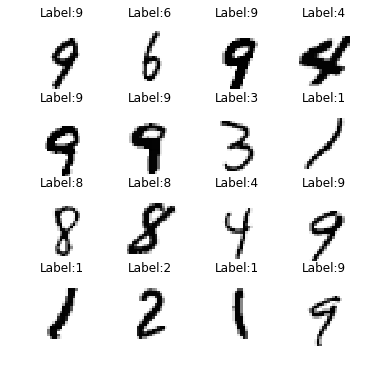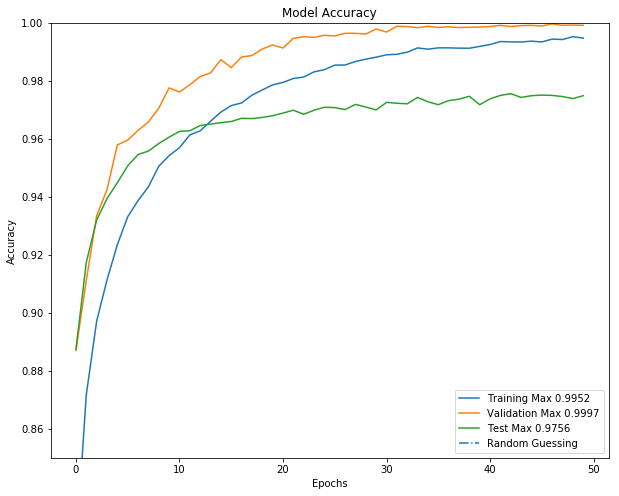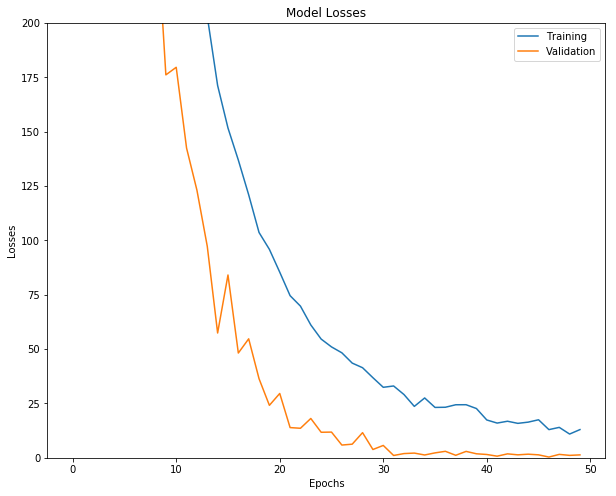# Tensorflow Deep Neural Network Logistic Regression on MNIST

Posted 2018-10-21

### Goal:¶

Build a deep neural network (in this case 1 hidden layer beyond logistic regression) using TensorFlow to classify handwritten digits [0, 1, 2, 3, 4, 5, 6, 7, 8, 9]

Performance benchmarks of different approaches: https://en.wikipedia.org/wiki/MNIST_database#Classifiers

Reproduction from AIND Deep Learning: https://www.udacity.com/course/deep-learning-nanodegree--nd101

In :
import math

import matplotlib.image as mpimg
import matplotlib.pyplot as plt
import numpy as np
from sklearn.model_selection import KFold
import tensorflow as tf
from tqdm import tqdm_notebook as tqdm

%matplotlib inline

/anaconda3/envs/aind-dl/lib/python3.5/importlib/_bootstrap.py:222: RuntimeWarning: compiletime version 3.6 of module 'tensorflow.python.framework.fast_tensor_util' does not match runtime version 3.5
return f(*args, **kwds)


### Import MNIST dataset¶

In :
def load_data():
"""Gets MNIST data. https://www.tensorflow.org/api_docs/python/tf/keras/datasets/mnist

Returns:
X_train (ndarray): training set data
Y_train (ndarray): training set ground truth labels
X_test (ndarray): test set data
Y_test (ndarray): test set ground truth labels
"""
mnist = tf.keras.datasets.mnist
(X_train, Y_train), (X_test, Y_test) = mnist.load_data()
print('-----Training Set Dimensions-----')
print(X_train.shape)
print(Y_train.shape)
print('\n-----Test Set Dimensions-----')
print(X_test.shape)
print(Y_test.shape)
return X_train, Y_train, X_test, Y_test

X_train, Y_train, X_test, Y_test = load_data()

-----Training Set Dimensions-----
(60000, 28, 28)
(60000,)

-----Test Set Dimensions-----
(10000, 28, 28)
(10000,)


### Samples of the 28x28 input data¶

In :
def show_sample_data(rows, cols):
"""Visualizes individual sample observerations in a grid.

Args:
rows (int): rows in grid
cols (int): columns in grid
"""
fig, axes = plt.subplots(rows, cols, figsize=(rows * 1.5, cols * 1.5))
for i in range(rows):
for j in range(cols):
idx = np.random.randint(len(Y_train))
axes[i,j].imshow(X_train[idx], cmap='Greys')
axes[i,j].set_title(('Label:{:d}'.format(Y_train[idx])))
axes[i,j].set_axis_off()

show_sample_data(4, 4)### Preprocess the data¶

In :
input_dim = X_train.flatten().shape
n_classes = len(np.unique(Y_train))

# Flatten 2D input data X_train and X_test into 1D vector
X_train = X_train.reshape([-1, input_dim])
X_test = X_test.reshape([-1, input_dim])

# Transform labels Y_train and Y_test to one hot encoding
Y_train_onehot = np.zeros([Y_train.shape, n_classes])
for i in range(Y_train.shape):
Y_train_onehot[i][Y_train[i]] = 1
Y_train = Y_train_onehot

Y_test_onehot = np.zeros([Y_test.shape, n_classes])
for i in range(Y_test.shape):
Y_test_onehot[i][Y_test[i]] = 1
Y_test = Y_test_onehot

print(X_train.shape, X_test.shape, Y_train.shape, Y_test.shape)

(60000, 784) (10000, 784) (60000, 10) (10000, 10)


### Build Neural Network Model¶In :
# Model Hyperparameters
learning_rate = 0.001
layer1_hidden_units = 800
train_keep_rate = 0.8
test_keep_rate = 1.0

In :
tf.reset_default_graph()

# Training data / label ground truth placeholders
X = tf.placeholder(tf.float32, [None, input_dim], name='input_X')
Y = tf.placeholder(tf.float32, [None, n_classes], name='output_Yhat')
keep_rate = tf.placeholder(tf.float32)

# Weights to train
w = {
'layer_1_w': tf.Variable(tf.truncated_normal([input_dim, layer1_hidden_units]), name='layer_1_w'),
'layer_2_w': tf.Variable(tf.truncated_normal([layer1_hidden_units, n_classes]), name='layer_2_w'),
}
b = {
'layer_1_b': tf.Variable(tf.truncated_normal([layer1_hidden_units]), name='layer_1_b'),
'layer_2_b': tf.Variable(tf.truncated_normal([n_classes]), name='layer_2_b'),
}


### Build Neural Network Graph¶

In :
# Training Hyperparameters
batch_size = 128
epochs = 50
display_epoch_step = 5
kFolds = 5

In :
# Build the graph
with tf.name_scope('layer_1'):
layer_1 = tf.nn.relu(layer_1, name='layer_1_relu')
layer_1 = tf.nn.dropout(layer_1, keep_rate)

with tf.name_scope('layer_2'):

In :
# Loss function
train_loss = tf.reduce_mean(tf.nn.softmax_cross_entropy_with_logits_v2(labels=Y, logits=Yhat_logits),
name='train_loss')

In :
# Optimizer


### Helper Functions¶

In :
def _accuracy():
"""Calculate accuracy based on Yhat_logits.

Returns:
(float): ratio of values that matched ground truth labels
"""
correct_predictions = tf.equal(tf.argmax(Yhat_logits, axis=1), tf.argmax(Y, axis=1))
return tf.reduce_mean(tf.cast(correct_predictions, tf.float32))

In :
def _cross_validate(X_train, Y_train, kFolds):
"""Generator that splits training set into training and validation.  Done via cross-validation.

Args:
X_train (ndarray): training data
Y_train (ndarray): training labels
kFolds (int): how many folds to split the data set into

Yields:
X_train[train_idx] (ndarray): training data fold
Y_train[train_idx] (ndarray): training label fold
X_train[test_idx] (ndarray): validation data fold
Y_train[test_idx] (ndarray): validation label fold
"""
kf = KFold(n_splits=kFolds)
for train_idx, test_idx in kf.split(X_train):
yield X_train[train_idx], Y_train[train_idx], X_train[test_idx], Y_train[test_idx]

In :
def _batched(X_train, Y_train, batch_size):
"""Generators that splits training data into batches.

Args:
X_train (ndarray): training data
Y_train (ndarray): training labels
batch_size (int): batch size

Yields:
X_train[idx:idx+batch_size] (ndarray): next batch of training data
Y_train[idx:idx+batch_size] (ndarray): next batch of training label data
"""
idx = 0
while idx < len(X_train):
if len(X_train) - idx < batch_size:
yield X_train[idx:], Y_train[idx:]
else:
yield X_train[idx:idx+batch_size], Y_train[idx:idx+batch_size]
idx += batch_size


### Train the Model (main loop)¶

In :
# Run the model
init = tf.global_variables_initializer()
sess = tf.InteractiveSession()
sess.run(init)

model_train_losses = []
model_train_acc = []
model_valid_losses = []
model_valid_acc = []
model_test_acc = []

for epoch in tqdm(range(epochs)):
# Split training data into KFolds
if epoch % kFolds == 0:
kFolded = _cross_validate(X_train, Y_train, kFolds)
X_train_fold, Y_train_fold, X_valid_fold, Y_valid_fold = next(kFolded)

# Train using batches
total_batches = math.ceil(X_train_fold.shape // batch_size)
batched_data = _batched(X_train_fold, Y_train_fold, batch_size)
for batch in range(total_batches):
batch_X_train, batch_Y_train = next(batched_data)
sess.run(optimizer, feed_dict={X: batch_X_train, Y: batch_Y_train, keep_rate: train_keep_rate})

# Record model performance at each epoch
e_train_loss, e_train_acc = sess.run([train_loss, _accuracy()], feed_dict={X: X_train, Y: Y_train, keep_rate: train_keep_rate})
e_valid_loss, e_valid_acc = sess.run([train_loss, _accuracy()], feed_dict={X: X_valid_fold, Y: Y_valid_fold, keep_rate: test_keep_rate})
e_test_acc = sess.run(_accuracy(), feed_dict={X: X_test, Y: Y_test, keep_rate: test_keep_rate})
model_train_losses.append(e_train_loss)
model_train_acc.append(e_train_acc)
model_valid_losses.append(e_valid_loss)
model_valid_acc.append(e_valid_acc)
model_test_acc.append(e_test_acc)

if epoch % display_epoch_step == 0:
# Display during training
print('-----Epoch: {}-----'.format(epoch+1))
print('tr_loss\t\t tr_acc \t v_loss\t\t v_acc\t\t test_acc')
print('{0}\t {1:.4f}\t\t {2:.4f}\t {3:.4f}\t\t {4:.4f}\t\t'
.format(str(e_train_loss), e_train_acc, e_valid_loss, e_valid_acc, e_test_acc))

sess.close()

-----Epoch: 1-----
tr_loss		 tr_acc 	 v_loss		 v_acc		 test_acc
3976.0513	 0.8179		 2230.8972	 0.8870		 0.8874
-----Epoch: 6-----
tr_loss		 tr_acc 	 v_loss		 v_acc		 test_acc
800.1309	 0.9331		 476.3055	 0.9596		 0.9507
-----Epoch: 11-----
tr_loss		 tr_acc 	 v_loss		 v_acc		 test_acc
327.8263	 0.9570		 179.5950	 0.9762		 0.9626
-----Epoch: 16-----
tr_loss		 tr_acc 	 v_loss		 v_acc		 test_acc
151.67596	 0.9715		 84.0754	 0.9846		 0.9660
-----Epoch: 21-----
tr_loss		 tr_acc 	 v_loss		 v_acc		 test_acc
85.37141	 0.9795		 29.5893	 0.9913		 0.9689
-----Epoch: 26-----
tr_loss		 tr_acc 	 v_loss		 v_acc		 test_acc
50.999863	 0.9854		 11.8225	 0.9955		 0.9708
-----Epoch: 31-----
tr_loss		 tr_acc 	 v_loss		 v_acc		 test_acc
32.42161	 0.9890		 5.6869	 0.9968		 0.9726
-----Epoch: 36-----
tr_loss		 tr_acc 	 v_loss		 v_acc		 test_acc
23.166948	 0.9914		 2.2607	 0.9984		 0.9718
-----Epoch: 41-----
tr_loss		 tr_acc 	 v_loss		 v_acc		 test_acc
17.396715	 0.9926		 1.5225	 0.9987		 0.9738
-----Epoch: 46-----
tr_loss		 tr_acc 	 v_loss		 v_acc		 test_acc
17.490263	 0.9934		 1.3632	 0.9989		 0.9751



### Visualize Performance¶

In :
plt.figure(figsize=(10,8))
plt.plot(model_train_acc, label='Training Max {0:.4f}'.format(max(model_train_acc)))
plt.plot(model_valid_acc, label='Validation Max {0:.4f}'.format(max(model_valid_acc)))
plt.plot(model_test_acc, label='Test Max {0:.4f}'.format(max(model_test_acc)))
plt.axhline(y=0.1, label='Random Guessing', linestyle='-.')

plt.title('Model Accuracy')
plt.xlabel('Epochs')
plt.ylabel('Accuracy')
plt.ylim([0.85,1])
plt.legend()

Out:
<matplotlib.legend.Legend at 0x1a2b73d550>In :
plt.figure(figsize=(10,8))
plt.plot(model_train_losses, label='Training')
plt.plot(model_valid_losses, label='Validation')

plt.title('Model Losses')
plt.xlabel('Epochs')
plt.ylabel('Losses')
plt.ylim([0, 200])
plt.legend()

Out:
<matplotlib.legend.Legend at 0x1a2b75af98>### Results¶

• Though MNIST is an easy data set (all observations are the same size input, centered, greyscale, no weird orientations), we managed to achieve <2.4% error with just 1 hidden layer, which is just a little off the 1.6% error rate recorded here for 2 layer NN. https://en.wikipedia.org/wiki/MNIST_database#Classifiers
• Note that by flattening the 2D vector into 1D we lose relationships in 2D space
• Dropouts really help with regularization
In [ ]: